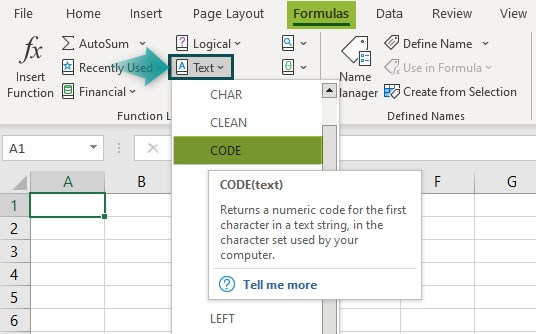# CODE Excel FunctionArticle byExcelMojo Team## What Is CODE Function In Excel?

The CODE Function in Excel finds the ASCII code for the selected character in a given dataset. The input character can be an alphabet, number, symbol, etc., and then the function returns the numeric code. ASCII stands for American Standard Code for Information Interchange.

The CODE Excel function is a built-in function, so we can insert the formula from the “Function Library” or enter it directly in the worksheet. For example, we will find the ASCII value of the given values using the CODE Function.

Select cell B2, enter the formula CODE(A2), and press “Enter”.

The output is shown above.

###### Key Takeaways
• The CODE Excel Function’s main purpose is to return the ASCII code of a character of the first character in any cell.
• The function always gives the numeric value as a result, as they are pre-set permanently.
• The output is different on different operating systems, i.e., the Windows OS uses the ANSI character set, and MAC-OS uses the Macintosh character set.
• Irrespective of the selected value’s character length, the CODE function will always give the ASCII code of only the first character.

### CODE() Excel Formula

The syntax of the CODE Excel formula is,

The argument of the CODE Excel formula is,

• text: It is a mandatory argument. It is the selected value to find the ASCII code of the first character, irrespective of the character length.

### How To Use CODE Excel Function?

We can use the CODE Excel function in 2 methods, namely,

1. Access from the Excel ribbon.
2. Enter in the worksheet manually.

#### Method #1 – Access from the Excel ribbon

First, choose an empty cell – select the “Formulas” tab – go to the “Function Library” group – click the “Text” option drop-down – select the “CODE” function, as shown below.

The “Function Arguments” window appears. Enter the argument value in the “Text” field – click “OK”, as shown below.

#### Method #2 – Enter in the worksheet manually

1. Select an empty cell for the output.
2. Type =CODE( in the selected cell. [Alternatively, type “=C” and double-click the CODE function from the list of suggestions shown by Excel.]
3. Enter the arguments as cell values or cell references in Excel.
4. Close the brackets and press the “Enter” key.

Let us take an example to understand this function.

We will calculate the ASCII code of the given data using CODE Excel function.

In the table, the data is,

• Column A: contains Text.
• Column B: contains Output.

The steps to find the ASCII code using CODE Excel function are,

1. Select cell B2 and enter the formula =CODE(A2).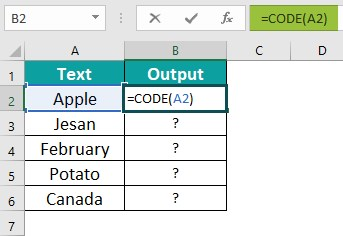2. Press the “Enter” key. The result is 65, as shown below.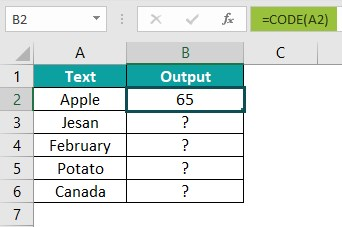3. Drag the formula from cell B2 to B6 using the fill handle.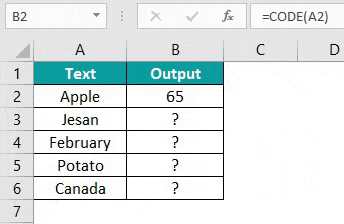The output is shown above.

### Examples

We will understand some advanced scenarios using the CODE Excel function examples.

#### #Example 1

We will calculate the ASCII code for the set numeric values using the CODE Function.

In the table, the data is,

• Column A: contains Text Value as Numbers.
• Column B: contains Output.

The steps to find the code using the CODE function are,

Step 1: Select cell B2, enter the formula =CODE(A2), and press “Enter”. The result is “49”, as shown below.

Step 2: Drag the formula from cell B2 to B6 using the fill handle. The output is shown below.

#### #Example 2

We will calculate the ASCII code of the set symbol values using the CODE Function.

In the table, the data is,

• Column A: contains Text Value as Symbols.
• Column B: contains Output.

The steps to find the code using the CODE Excel function are,

Step 1: Select cell B2, enter the formula =CODE(A2), and press the “Enter” key. The result is “60”, as shown below.

Step 2: Drag the formula from cell B2 to B12 using the fill handle. The output is shown below.

#### #Example 3

We will calculate the ASCII code for the set string values using the CODE Excel Function.

In the table, the data is,

• Column A: contains Text as a string.
• Column B: contains Output.

The steps to find the ASCII code using the CODE Excel function are,

Step 1: Select cell B2, enter the formula =CODE(A2), and press the “Enter” key. The result is “87”, as shown below.

Step 2: Drag the formula from cell B2 to B3 using the fill handle. The output is shown below.

#### #Example 4

We will calculate the ASCII code for the set idioms values using the CODE Excel Function.

In the table, the data is,

• Column A: contains Text as Idioms.
• Column B: contains Output.

The steps to find code using the CODE Excel function are,

Step 1: Select cell B2, enter the formula =CODE(A2), and press the “Enter” key. The result is “80”, as shown below.

2: Drag the formula from cell B2 to B6 using the fill handle. The output is shown below.

### Important Things To Note

• The #VALUE! error occurs when the selected cell value is blank or empty.
• The #NAME? error occurs when no argument is entered.
• The CODE function is an Excel Text function in the Function Library group with one mandatory argument, i.e., text, which can be an alphabet, symbol, text, sentence, numbers, etc.

What does the CODE function do in Excel?

The CODE Function is an Excel Text function in the Function Library group. The function fetches the preset numeric code of the selected value, irrespective of the characters length.
The syntax of the CODE function is =CODE(text)

How does the CODE function work in Excel?

The CODE function works by following the below steps;
1. Select an empty cell for the output.
2. Type =CODE( in the selected cell. [Alternatively, type “=C” and double-click the CODE function from the list of suggestions shown by Excel.]
3. Enter the arguments as cell values or cell references.
4. Close the brackets and press the “Enter” key.

For example, we will calculate the ASCII code using the CODE Excel function are,

Select cell B2, enter the formula =CODE(A2), press the “Enter” key, and drag the formula from cell B2 to B3 using the fill handle. The output is shown below.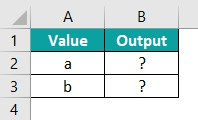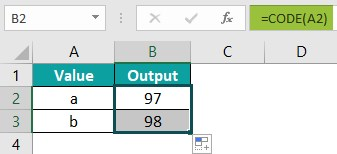Where is the CODE Function in Excel?

One can activate the CODE Function in Excel using the following steps:

First, choose an empty cell – select the “Formulas” tab – go to the “Function Library” group – click the “Text” option drop-down – select the “CODE” function, as shown below.